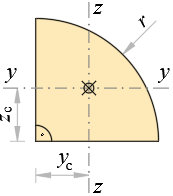### Geometrical Properties of Quarter CircleArea

A = π·r24 m2

Centroid

zc = yc = rπ m

Perimeter

P = (π2 + 2)·r m

Second moments of area

Iz = Iy = (π164π)·r4 m4

Iyz = (184π)·r4 m4

Principal area moments

I1 = Iy + abs(Iyz) m4

I2 = Iyabs(Iyz) m4

Angle of principal axis

α1 = atan(IyI1Iyz) о

Polar moment of area

Ix = 2·Iy m4